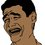# A way to determine the cubic formula

Hi everyone, some time ago I was playing with Cardano's method to solve the general cubic equation. Then I discovered a new way to determine the same formula, altough is a little longer (also because I wanted to detail every step I do). It is very similar to the method of Lagrange, with some differences. That method finds $u^3+v^3$ and $uv$, while I find $u$ and $v$ directly, and I also determine an expression for the discriminant. Here it goes:

Let's start with the equation we want to solve: $x^3+bx^2+cx+d=0$ where $b,c,d$ are complex numbers. Let its roots be $x_1,x_2,x_3$. By Vieta's formulas we know that:

$x_1+x_2+x_3=-b \\ x_1x_2+x_1x_3+x_2x_3=c \\ x_1x_2x_3=-d$

Then, let $\Delta$ be the discriminant of the equation and $w$ a primitive cube root of unity. Then, by the definition of discriminant we have:

$\Delta=((x_1-x_2)(x_2-x_3)(x_3-x_1))^2$

Expand it to obtain something interesting:

$\Delta=((x_1x_2^2+x_2x_3^2+x_3x_1^2)-(x_1^2x_2+x_2^2x_3+x_3^2x_1))^2$

Now, let $m=x_1x_2^2+x_2x_3^2+x_3x_1^2$ and $n=x_1^2x_2+x_2^2x_3+x_3^2x_1$. So, $\Delta=(m-n)^2$. Our first objective is to find $m$ and $n$ in terms of the coefficients. The trick is first find $m+n$ and $mn$:

$m+n=x_1x_2^2+x_2x_3^2+x_3x_1^2+x_1^2x_2+x_2^2x_3+x_3^2x_1\\ m+n=x_1x_2(x_1+x_2)+x_2x_3(x_2+x_3)+x_3x_1(x_3+x_1) \\ m+n=x_1x_2(x_1+x_2+\color{#D61F06}{x_3})+x_2x_3(\color{#D61F06}{x_1}+x_2+x_3)+x_3x_1(x_3+x_1+\color{#D61F06}{x_2})-\color{#D61F06}{3x_1x_2x_3} \\ m+n=(x_1+x_2+x_3)(x_1x_2+x_1x_3+x_2x_3)-3x_1x_2x_3 \\ \boxed{m+n=-bc+3d}$

$mn=(x_1x_2^2+x_2x_3^2+x_3x_1^2)(x_1^2x_2+x_2^2x_3+x_3^2x_1) \\ mn=\color{#3D99F6}{(x_1x_2)^3+(x_1x_3)^3+(x_2x_3)^3}+3(x_1x_2x_3)^2+x_1x_2x_3(\color{#D61F06}{x_1^3+x_2^3+x_3^3}) \\ mn=\color{#3D99F6}{(x_1x_2+x_1x_3+x_2x_3)^3-3(x_1+x_2+x_3)(x_1x_2+x_1x_3+x_2x_3)(x_1x_2x_3)+3(x_1x_2x_3)^2}+3(x_1x_2x_3)^2+x_1x_2x_3(\color{#D61F06}{(x_1+x_2+x_3)^3-3(x_1+x_2+x_3)(x_1x_2+x_1x_3+x_2x_3)+3x_1x_2x_3}) \\ mn=c^3-3bcd+3d^2+3d^2-d(-b^3+3bc-3d)\\ mn=c^3-3bcd+3d^2+3d^2+b^3d-3bcd+3d^2 \\ \boxed{mn=c^3-6bcd+9d^2+b^3d}$

Now, with the identity $(m-n)^2=(m+n)^2-4mn$ we can find $\Delta$, and hence $m-n$:

$\Delta=(-bc+3d)^2-4(c^3-6bcd+9d^2+b^3d)\\ \Delta=b^2c^2-6bcd+9d^2-4c^3+24bcd-36d^2-4b^3d \\ \boxed{\Delta=b^2c^2-4c^3-4b^3d+18bcd-27d^2}$

WLOG assume that $m>n$, then:

$m-n=\sqrt{\Delta}$

Easily we can find $m$ and $n$:

$\boxed{m=\dfrac{-bc+3d+\sqrt{\Delta}}{2}} \quad \boxed{n=\dfrac{-bc+3d-\sqrt{\Delta}}{2}}$

Now, our second objetive is try to find the roots in terms of the coefficients. Let's introduce $u$ and $v$:

$u=x_1+x_2w^2+x_3w \\ v=x_1+x_2w+x_3w^2$

Notice that $uw=x_1w+x_2+x_3w^2$ and $vw=x_1w+x_2w^2+x_3$, similarly $uw^2=x_1w^2+x_2w+x_3$ and $vw^2=x_1w^2+x_2+x_3w$. We can sum them in 9 different ways, but only 3 produce results independent of $w$, they are:

$u+v=2x_1-x_2-x_3 \\ uw+vw^2=-x_1+2x_2-x_3 \\ uw^2+vw=-x_1-x_2+2x_3$

From here, see how we can obtain the roots. Yes, simply adding $-b$ to each equation and dividing by 3 does the work:

$x_1=\dfrac{u+v-b}{3} \\ x_2=\dfrac{uw+vw^2-b}{3} \\ x_3=\dfrac{uw^2+vw-b}{3}$

Then, our last objective is to determine $u$ and $v$. From $u$, cube both sides:

$u^3=\color{#D61F06}{x_1^3+x_2^3+x_3^3}+3(\color{#20A900}{x_1^2x_2w^2}+\color{#3D99F6}{x_1x_2^2w}+\color{#20A900}{x_2^2x_3w^2}+\color{#3D99F6}{x_2x_3^2w}+\color{#20A900}{x_3^2x_1w^2}+\color{#3D99F6}{x_3x_1^2w})+6x_1x_2x_3\\ u^3=\color{#D61F06}{(x_1+x_2+x_3)^3-3(x_1+x_2+x_3)(x_1x_2+x_1x_3+x_2x_3)+3x_1x_2x_3}+3(\color{#20A900}{nw^2}+\color{#3D99F6}{mw})+6x_1x_2x_3 \\ u^3=-b^3+3bc-3d+3\left(\dfrac{-bc+3d-\sqrt{\Delta}}{2}w^2+\dfrac{-bc+3d+\sqrt{\Delta}}{2}w\right)-6d \\ u^3=-b^3+3bc-9d+\dfrac{(-3bc+9d)(w^2+w)+3\sqrt{\Delta}(w-w^2)}{2}$

Remember that $w^2+w=-1$ and $w-w^2=\sqrt{3}i$, then:

$u^3=\dfrac{-2b^3+6bc-18d+3bc-9d+3\sqrt{3}\sqrt{\Delta}i}{2} \\ \boxed{u=\sqrt{\dfrac{-2b^3+9bc-27d+\sqrt{-27\Delta}}{2}}}$

In a similar way we can obtain $v$:

$\boxed{v=\sqrt{\dfrac{-2b^3+9bc-27d-\sqrt{-27\Delta}}{2}}}$

We obtained back $u$ and $v$ by taking cube root in both sides. We don't worry about the three possible cube roots, because we worked on that when we combined $u$ and $v$ to form the roots.

If we plug in everything, we obtain the messy formulas for the roots, this is one of them:

$x_1=\dfrac{\sqrt{\dfrac{-2b^3+9bc-27d+\sqrt{-27\Delta}}{2}}+\sqrt{\dfrac{-2b^3+9bc-27d-\sqrt{-27\Delta}}{2}}-b}{3}$

Bonus question: the expression inside the square root, $-27\Delta$ can be written as $\Delta_1^2-4\Delta_2^3$. Find $\Delta_1$ and $\Delta_2$.

I hope that you liked this note, it's my first note in Brilliant :D If you think that some steps could have been simplified, feel free to tell me.Note by Alan Enrique Ontiveros Salazar
5 years, 9 months ago

This discussion board is a place to discuss our Daily Challenges and the math and science related to those challenges. Explanations are more than just a solution — they should explain the steps and thinking strategies that you used to obtain the solution. Comments should further the discussion of math and science.

When posting on Brilliant:

• Use the emojis to react to an explanation, whether you're congratulating a job well done , or just really confused .
• Ask specific questions about the challenge or the steps in somebody's explanation. Well-posed questions can add a lot to the discussion, but posting "I don't understand!" doesn't help anyone.
• Try to contribute something new to the discussion, whether it is an extension, generalization or other idea related to the challenge.

MarkdownAppears as
*italics* or _italics_ italics
**bold** or __bold__ bold
- bulleted- list
• bulleted
• list
1. numbered2. list
1. numbered
2. list
Note: you must add a full line of space before and after lists for them to show up correctly
paragraph 1paragraph 2

paragraph 1

paragraph 2

[example link](https://brilliant.org)example link
> This is a quote
This is a quote
    # I indented these lines
# 4 spaces, and now they show
# up as a code block.

print "hello world"
# I indented these lines
# 4 spaces, and now they show
# up as a code block.

print "hello world"
MathAppears as
Remember to wrap math in $$ ... $$ or $ ... $ to ensure proper formatting.
2 \times 3 $2 \times 3$
2^{34} $2^{34}$
a_{i-1} $a_{i-1}$
\frac{2}{3} $\frac{2}{3}$
\sqrt{2} $\sqrt{2}$
\sum_{i=1}^3 $\sum_{i=1}^3$
\sin \theta $\sin \theta$
\boxed{123} $\boxed{123}$

Sort by:

I am tempted to appreciate your patience in typing this out with $\LaTeX$. Thank you !

- 5 years, 9 months ago

This is great. The formula for $x_1$ is already ginormous, I can't imagine how $x_2$ and $x_3$ will look like even after simplifying.

Now do the quartic formula ahahahaha

- 5 years, 9 months ago

For $x_2$ and $x_3$ we just have to add some $w$'s. For the quartic formula there is a similar approach, let me try to do it without die trying.

- 5 years, 9 months ago

I think the analogy would be that Your Approach vs Cardano's is like Chemistry vs Alchemy. Very nice!

This is the kind of thing that, although very long, I probably could still [eventually] remember how it could be done. Cardano's? I could never remember his way.

- 5 years, 9 months ago

Great job!

- 5 years, 9 months ago

Nice work. Appreciate it.

- 5 years, 8 months ago

Whoa !! You PLAYED well !!

- 5 years, 9 months ago

A great thanks. This is very helpful :)

- 5 years, 9 months ago

This is very cool!

- 5 years, 9 months ago

i dont understand since i am still a highschool student

- 5 years, 9 months ago

We all are high school students bro :P

- 5 years, 9 months ago